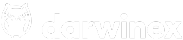CFDs are complex instruments and come with a high risk of losing money rapidly due to leverage. 66 % of retail investor accounts lose money when trading CFDs with this provider. You should consider whether you understand how CFDs work and whether you can afford to take the high risk of losing your money.# Ra trades information structure?

Hi!

So I managed to obtain the information for Risk Adjustment IA, but for some reason, I’m struggling to understand the structure of trades information.

`item -> (long) Millis item -> (double) D-Periods item -> (double) Leverage target of day (deprecated) item -> (double) VaR of day item[\$position] -> (long) Open time of position item[\$position] -> (long) Duration of position item[\$position] -> (double) D-Leverage of position item[\$position] -> (double) VaR adjustment of position item[\$position] -> (integer) Number of open trades item[\$position] -> (double) Return of position item[\$position] -> (double) Leverage target of position`

for item I see a list of rows such as for example:
`15.643229 0.139179 [[1519301008000, 19000, 13.3370734994832, 0.0,... 9.764229 0.241560 [[1529604051000, 54755999, 3.137995494210046, ... NaN NaN NaN 9.266590 0.230258 [[1530127247000, 41000, 3.2132923750775437, 0....`

First two values being deprecated leverage, and VaR of day.

Just in case, this is the code to obtain the matrix I’m talking about:
`def get_h_ra(self, name=None): # get historical Ra values url = self.base_url + "/products/{}/history/riskadjustment".format(name) resp = json.loads(requests.get(url, headers=self.base_headers).text) # obtain API response df = pd.DataFrame(resp) # convert to pandas Dataframe aux1 = df.iloc[:, 2].apply(pd.Series) # Get historical positions print(aux1) return aux1`

Could you let me know what the list of positions represents? Are there positions for one day?

Also, if anyone could help me out on how to transform this info into a matrix it would be much appreciated. (Not a developer myself, sorry)

Well, it seems I reached a quite inefficient solution.

First, each row are positions for a day, good API documentation.

The whole Python method would be something like this:

``````def get_h_ra(self, name=None):
if name is None:
name = self.name
url = self.base_url + "/products/{}/history/riskadjustment".format(name)
resp = json.loads(requests.get(url, headers=self.base_headers).text)
df = pd.DataFrame(resp)
aux = df.iloc[:, 2].values
out = []  # below lies the "magic"
for x in aux:
if x:  # each row. Avoid null values
for y in x:  # third value is the list of trades
if y:  # Avoid null values again
out.append(y)
df = pd.DataFrame(out,
columns=["OpenDate", "Duration (ms)", "D-Leverage",
"VaR Adjust", "No. trades", "Return",
"Leverage tgt"])
df["OpenDate"] = pd.to_datetime(df["OpenDate"], unit="ms")
df.set_index("OpenDate", inplace=True)
return df``````